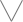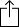C♭
D♭
E♭
F♭
G♭
A♭
B♭
Chord
Scale
Custom
3nps
CAGED
None
Notes
Degrees
Intervals
None

1
2
3
4
5
1
2
3
4
5
6
7
8
9
10
11
12
13
14
15
16
17
18
19
20
21
22
23
24
E
B
G
D
A
E
B
F
G
G
A
A
B
B
C
D
D
E
E
F
G
G
A
A
B
B
C
D
D
E
E
C
D
D
E
E
F
G
G
A
A
B
B
C
D
D
E
E
F
G
G
A
A
B
B
A
A
B
B
C
D
D
E
E
F
G
G
A
A
B
B
C
D
D
E
E
F
G
G
E
E
F
G
G
A
A
B
B
C
D
D
E
E
F
G
G
A
A
B
B
C
D
D
B
B
C
D
D
E
E
F
G
G
A
A
B
B
C
D
D
E
E
F
G
G
A
A
F
G
G
A
A
B
B
C
D
D
E
E
F
G
G
A
A
B
B
C
D
D
E
E
C
D
D
E
E
F
G
G
A
A
B
B
C
D
D
E
E
F
G
G
A
A
B
B

# Eb Egyptian Suspended for 7 String Guitar - CAGED Chart

Eb Egyptian suspended is a pentatonic scale. Pentatonic scales have 5 notes, "penta" comes from the greek word "Pentateuch" which means 5.

The Eb Egyptian suspended scale is derived from the Eb Dorian mode by taking scale degree 1, 2, 4, 5 and 7 of the scale.

E
F
A
B
D
EW
m3
W
m3
W
Play scaleand then